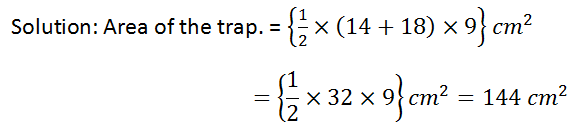# RS Aggarwal Class 8 Math Eighteenth Chapter Area of a Trapezium and a Polygon Exercise 18C Solution

## EXERCISE 18C

### OBJECTIVE QUESTIONS

#### Tick (√) the correct answer in each of the following:

(1) The parallel sides of a trapezium measure 14 cm and 18 cm and the distance between them is 9 cm. The area of the trapezium is

Ans: (b) 144 cm2(2) The lengths of the parallel sides of trapezium are 19 cm and 13 cm and its area is 128 cm2. The distance between the parallel sides is

Ans: (c) 8 cm

Solution: Let the distance be x cm.(3) The parallel sides of a trapezium are in the ratio 3 : 4 and the perpendicular distance between them is 12 cm. If the area of the trapezium is 630 cm2, then its shorter of the parallel sides is

Ans: (a) 45 cm

Solution: Let the length of the parallel sides are 3x cm and 4x cm.Hence, the lengths of the parallel sides are 45 cm and 60 cm.

Then, a shorter parallel side is 45 cm.

(4) The area of a trapezium is 180 cm2 and its height is 9 cm. If one the parallel side is longer than the other by 6 cm, the length of the longer of the parallel sides is

Ans: (b) 23 cm

Solution: Let the lengths of the parallel sides are x cm and (x + 6) cm.Then, the longer side is (17+6) = 23 cm

(5) In the given figure, AB DC and DA AB. If DC = 7 cm, BC = 10 cm, AB = 13 cm and CL ⊥ AB, the area of trap. ABCD isAns: (c) 80 cm2Updated: May 30, 2022 — 2:36 pm